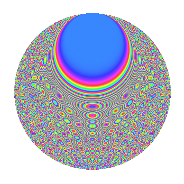# Properties

 Label 1890.2.btLevel 1890 Weight 2 Character orbit bt Rep. character $$\chi_{1890}(331,\cdot)$$ Character field $$\Q(\zeta_{9})$$ Dimension 576 Sturm bound 864

# Related objects

## Defining parameters

 Level: $$N$$ = $$1890 = 2 \cdot 3^{3} \cdot 5 \cdot 7$$ Weight: $$k$$ = $$2$$ Character orbit: $$[\chi]$$ = 1890.bt (of order $$9$$ and degree $$6$$) Character conductor: $$\operatorname{cond}(\chi)$$ = $$189$$ Character field: $$\Q(\zeta_{9})$$ Sturm bound: $$864$$

## Dimensions

The following table gives the dimensions of various subspaces of $$M_{2}(1890, [\chi])$$.

Total New Old
Modular forms 2640 576 2064
Cusp forms 2544 576 1968
Eisenstein series 96 0 96

## Trace form

 $$576q - 6q^{6} - 24q^{9} + O(q^{10})$$ $$576q - 6q^{6} - 24q^{9} - 24q^{11} + 6q^{14} + 48q^{17} + 24q^{21} - 36q^{23} - 6q^{29} + 72q^{33} - 6q^{36} + 12q^{39} + 12q^{41} - 6q^{45} - 36q^{47} - 36q^{49} + 72q^{51} + 72q^{54} + 6q^{56} + 48q^{57} + 120q^{59} - 18q^{61} + 96q^{62} + 36q^{63} - 288q^{64} + 12q^{65} + 108q^{69} + 18q^{70} - 48q^{71} - 144q^{73} + 72q^{74} + 60q^{77} - 96q^{78} + 36q^{79} + 12q^{80} - 24q^{81} + 6q^{84} - 72q^{85} + 48q^{86} + 120q^{87} - 72q^{91} - 36q^{92} + 48q^{93} + 36q^{94} + 48q^{98} + O(q^{100})$$

## Decomposition of $$S_{2}^{\mathrm{new}}(1890, [\chi])$$ into newform subspaces

The newforms in this space have not yet been added to the LMFDB.

## Decomposition of $$S_{2}^{\mathrm{old}}(1890, [\chi])$$ into lower level spaces

$$S_{2}^{\mathrm{old}}(1890, [\chi]) \cong$$ $$S_{2}^{\mathrm{new}}(189, [\chi])$$$$^{\oplus 4}$$$$\oplus$$$$S_{2}^{\mathrm{new}}(378, [\chi])$$$$^{\oplus 2}$$$$\oplus$$$$S_{2}^{\mathrm{new}}(945, [\chi])$$$$^{\oplus 2}$$

## Hecke Characteristic Polynomials

There are no characteristic polynomials of Hecke operators in the database